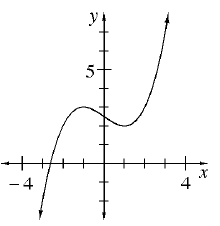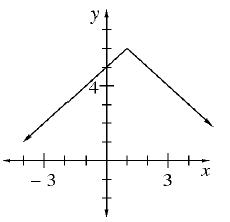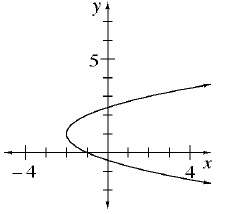### Home > AC > Chapter 11 > Lesson 11.1.5 > Problem11-63

11-63.

Which graphs below have a domain of all numbers? Which have a range of all numbers?

1.1.1.In which graph do all values of $x$ have a corresponding $y$-value?
Refer to the Math Notes box in Lesson 11.1.4.

Graphs (a) and (b)

In which graphs do all values of $y$ have a corresponding $x$-value?

Graphs (a) and (c)

A function can only have one $y$-value for each $x$-value.